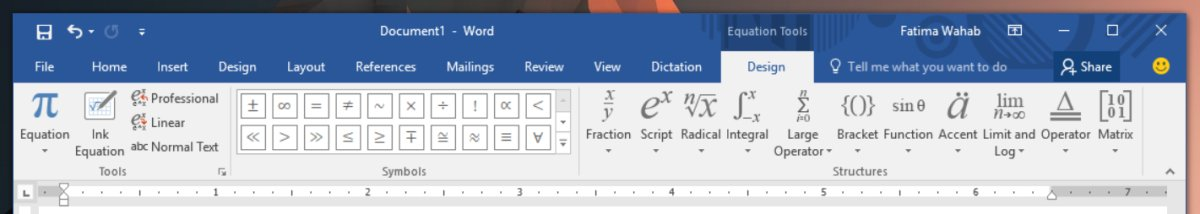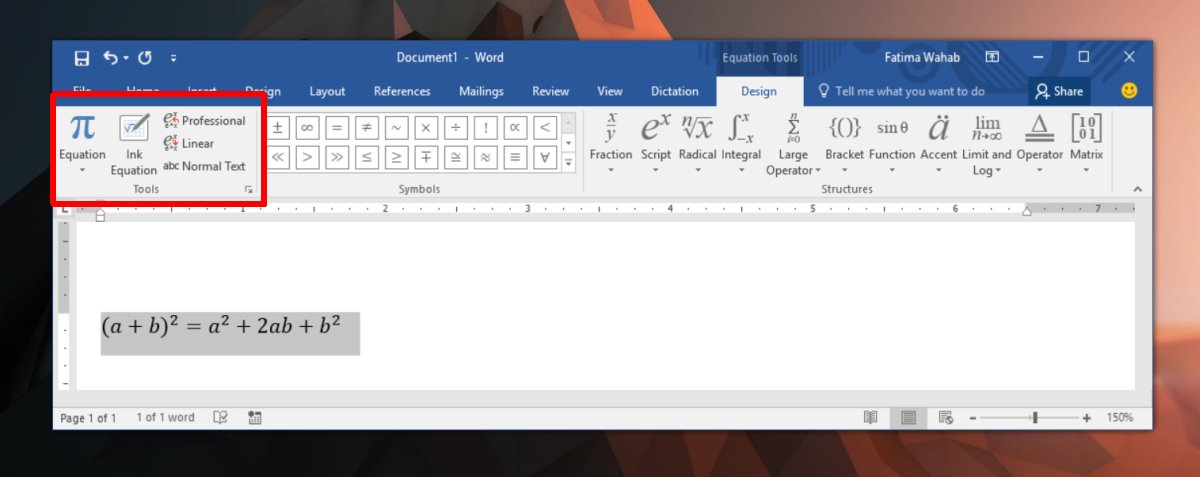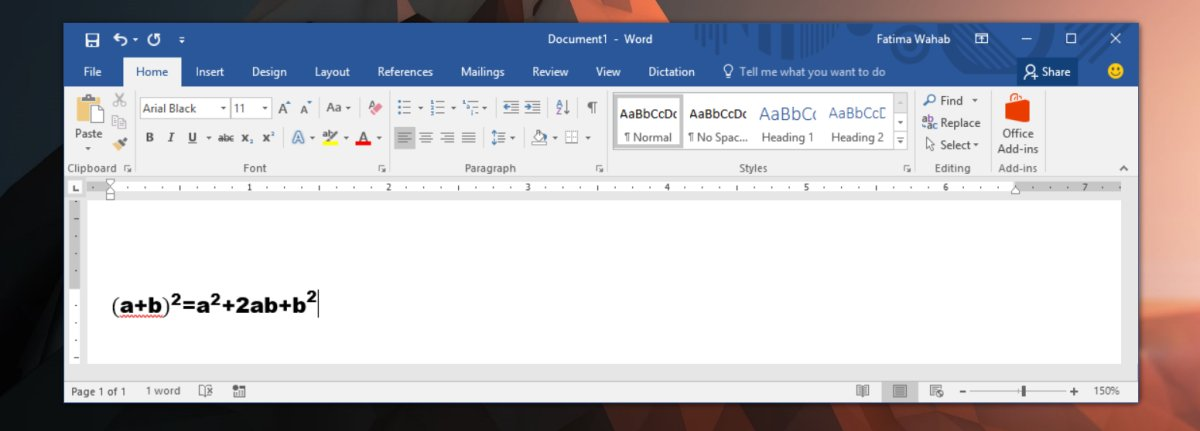# How To Change The Equation Font In MS Word

You can insert mathematical equations in MS Word. There is a dedicated tool for equations that supports both mathematical and statistical symbols. Additionally, there is a tool that lets you enter standard formulas for example, the formula for calculating the area of a circle. It really is very well equipped and on Windows 10, it supports Inking an equation. You can basically just write an equation with a graphical pen tool and MS Word will be able to read it. There is one limitation in the equation tool; it only has one font. If you change the equation font in MS Word via the Home tab on the ribbon, it changes back to the default maths font.

In truth, you can change the equation font in MS Word but it’s a slightly tricky process.

## Change The Equation Font In MS Word

First, you need to insert an equation. On the ribbon, go to Insert>Equation.Type in an equation. Once you’re done, select it and on the ‘Design’ tab, click the ‘Normal Text’ button on the Tools box.Next, go to the Home tab, and from the Font dropdown, select any font you like. The equation font will change.## Limitations

MS Word limits fonts available for mathematical equations for good reason. Fonts are generally developed for alphanumeric characters and some choice symbols that are commonly used. Very few fonts are developed with a full set of mathematical characters. When you choose to change the equation font in MS Word, you risk some characters not being available in said font.

For example, a font might not have statistical characters like the Sigma symbol or the under-root symbol in which case, you equation might not render fully. The default equation font in MS Word, Cambria Math, supports every character you need to insert an equation which is why most people stick to using it. It’s a very legible font and you can increase the text size or make it bold if you want to make it more legible.

If you’re determined to use a different font for equations in MS Word, look for a font that was built for mathematical input. There might not be that many fonts with a full mathematical character set but there are definitely some out there. Download and install the fonts on your PC the same way you would any other font and use the method described above to apply it to an equation. The equation editor doesn’t remember the font you used in your previous equation. You will have to change it for each one.

This site uses Akismet to reduce spam. Learn how your comment data is processed.